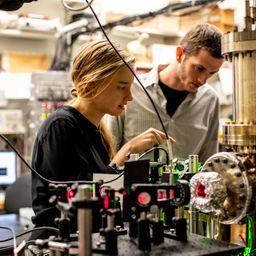Ask your homework questions to teachers and professors, meet other students, and be entered to win \$600 or an Xbox Series X 🎉Join our Discord!Numerade Educator

# The work function for platinum is 6.35 eV. (a) Convert the value of the work function from electron volts to joules. (b) Find the cutoff frequency for platinum. (c) What maximum wavelength of light incident on platinum releases photoelectrons from the platinum’s surface? (d) If light of energy 8.50 eV is incident on zinc, what is the maximum kinetic energy of the ejected photoelectrons? Give the answer in electron volts. (e) For photons of energy 8.50 eV, what stopping potential would be required to arrest the current of photoelectrons?

## a. 1.02 \times 10^{-18} Jb. 1.53 \times 10^{15} \mathrm{Hz}c. 196 \mathrm{nm}d. 2.15 eVe. 2.15 {V}

Quantum Physics

Atomic Physics

### Discussion

You must be signed in to discuss.
SB

Shreerup B.

December 2, 2020

SB

Shreerup B.

December 2, 2020

##### Top Physics 103 Educators
LB##### Marshall S.

University of Washington##### Aspen F.

University of Sheffield### Video Transcript

Okay, So when this problem we're given, the work function for platinum is 6.35 evey, and we want to convert it from evey to Jules. So, um, the convert from evey two Jews we do. Jules, we need to dis multiply by 1.6. So, too, because that's the conversion factor. That's how many electron volts there are, per jewel. So then you can get five is equal to go ahead and plug this into a calculator. Has explained 35 times, 1.6 and two times 10 to the minus in a team. And with that, I get one point recent conviction to do 3661 point. I'll just include a few extra, since I know we're gonna be adding and subtracting the rules are a little different. So 1.17 times 10 to the minus 18. Great. And then next what? We want the cutoff frequency. So for the coffer, Quincy, we want to say Okay, you're rejecting your shining light on platinum and then you rejecting the electrons. What's the absolute lowest energy? You could, um, shine. And that would be in the case that the electrons are ejected, but they can't move. They don't have any more energy. They've spent all their energy on ejection. So in that case, for, um, like in the general formula for the photoelectric effect, kinetic energy is energy of the photons minus fi. You can set that equal to zero, and then you can plug in for energy of the photons you get. So just kind of rearranging this again. He is Fi. And then he is equal to HC over Lambda. And then with that, let's see, what did we want, the frequency or the Oh, yeah. So I guess we could, so we'll probably get the wavelength later. Is that for? Well, no e I guess we don't have to. I was thinking we wanted to solve for wavelength, but I guess we just want to solve her frequency. So that's gonna be H f. And then if we saw for Africa, half is equal to fi over H. So I'm gonna take this fire that we've converted to a sigh and divided by h also an S I. So that's gonna be dividing by Planck's constant 6.2665 66. Sometime in my 34 and I got 1.5. Four times 10 of the 15. Great. And for C, we want to get the max wavelength of light. Um Oh! Ha ha. This was finding the Max leave, like Okay, great. What we want to do frequency is C over Lambda. So we can solve for Lambda. Lambda is equal to see Time's this thing inverted seven. I got that land eyes. He cool too, for plugging that into a calculator, inverting this frequency on multiplying by the speed of light at 1.95 times 10 to the minus seven. So 195 millimeters. Okay, now we're considering that if Light has 8.5 evey and its incident What ISS The kinetic energy of the rejected photo electrons. And then so for that one is the c e o d o k. This was D, Mrs C. And then Now what we want to do is D So we want to use this formula but not cross out the k e. So we can get the k e equals, um, the energy of the proton. So 8.5 e v minus the work function of zinc so that's minus 6.35 So let's see. That's 1.65 plus 0.5. So 1.15 TV Oh, sorry to 00.1 pipe and for party. Um, we want to get the stopping potential required to arrest the current of photo electrons. So, um, so he said, um, So you can also write this formula as setting an equal to, um from the charge of the electron times and applied voltage and if you want to read more about that, But, I mean, basically, you can turn the kinetic energy and potential energy by applying a voltage. And so that's just a on application of potential energy is charged times footage. So, um, so this is okay, so you can just set the secret of this and then you would not see so you would do this. And then, um, let's say you put these numbers in S i u nit. So you would take this and multiply these by e more than you would. Then they get V. You wouldn't. Yeah, a factor of you would be common to everything. And so, um, this answer turns out to be the same. So that's 2.15 volts.University of Washington

#### Topics

Quantum Physics

Atomic Physics

##### Top Physics 103 Educators
LB##### Marshall S.

University of Washington##### Aspen F.

University of Sheffield